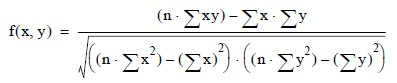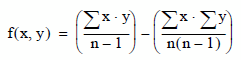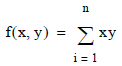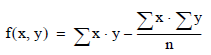Product
Release Number
5.4.6
Published
November 2018
Language
English (United States)
Last Update
2018-12-07
dita:mapPath
gxn1538171534877.ditamap
dita:ditavalPath
ft:empty
dita:id
B035-2301
Product Category
Software
The Matrix analysis will process the input data so that one of the following data reduction matrices can be exported via the Export Matrix analysis:
• Pearson-product moment correlations (COR)
• Covariances (COV)
• Sums of Squares and Cross-Products (SSCP)
• Corrected Sums of Squares and Cross-Products (CSSCP)
The formulas used to calculate these matrices are given below.
• Correlation — The Pearson Product-Moment Correlation value of the pairwise combinations of each column within the selected table. This is calculated as follows, for each pairwise combination of columns X and Y.where n is the total number of rows in the calculation.

• Covariance — The Covariance value of the pairwise combinations of each column within the selected table. This is calculated as follows, for each pairwise combination of columns X and Y.where n is the total number of rows in the calculation.

• Sums of Squares and Cross-Products — The Sums of Squares and Cross-Products value of the pairwise combinations of each column within the selected table. This is calculated as follows, for each pairwise combination of columns X and Y.where n is the total number of rows in the calculation.

• Corrected Sums of Squares and Cross-Products — The Corrected Sums of Squares and Cross-Products value of the pairwise combinations of each column within the selected table. This is calculated as follows, for each pairwise combination of columns X and Y.where n is the total number of rows in the calculation.

The Matrix functions must operate on numeric data. Columns of type DATE will not produce meaningful results.

By default, NULL values are handled via listwise deletion, but an option to effectively replace NULL values with zeros is also available. Listwise deletion means that if the value of any column to be included in the matrix is NULL, the entire row is omitted during matrix calculations.

If the Matrix UDF is not installed on the target Teradata database, the Matrix analysis has restart capabilities such that if a system failure occurs, or the Matrix analysis is stopped by the end-user, it can be restarted. When the interrupted Matrix analysis is re-executed, it will automatically begin its calculations at the point of stoppage.
The name of the Matrix analysis is used to fetch the matrix values from the database for those functions that are dependent upon a matrix, such as Export matrix.